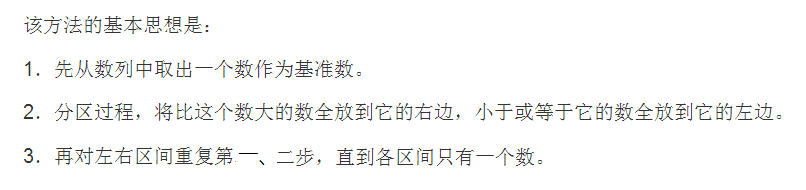## 快速排序_快速排序if (left < right) { mid = (right - left) / 2;_ZDF0414的博客-程序员秘密``````int GetMid(int a[], int left, int right)//三数取中
{
int mid = left + (right - left) / 2;
if (a[left] <a[right])
{
if (a[mid] < a[left])
{
return left;
}
else
{
if (a[mid]>a[right])
{
return right;
}
else
{
return mid;
}
}
}
else
{
if (a[right] > a[mid])
{
return right;
}
else
{
if (a[mid] > a[left])
{
return left;
}
else
{
return mid;
}
}
}
}

//递归实现
void Re_FastSort(int a[], int left, int right)
{
if (left >= right)
{
return;
}
if (right - left < 13)
{
InsertSort(a, right - left + 1);
return;
}
int begin = left;
int end = right;
int key = GetMid(a, left, right);
swap(a[left], a[key]);
int temp = a[left];
while (begin < end)
{
while (begin<end&&a[end]>temp)
{
end--;
}
if (begin < end)
{
a[begin] = a[end];
begin++;
}
while (begin<end&&a[begin]<temp)
{
begin++;
}
if (begin < end)
{
a[end] = a[begin];
end--;
}
}
a[begin] = temp;
Re_FastSort(a, left, begin - 1);
Re_FastSort(a, begin + 1, right);
}
``````

``````//栈实现
#include<stack>
void NoRe_FastSort(int a[], int left, int right)
{
assert(a);
struct left_right
{
int _left;
int _right;
left_right(int left, int right)
:_left(left)
, _right(right)
{}
};
stack<left_right>s;
s.push(left_right(left, right));
while (!s.empty())
{
int begin = s.top()._left;
int end = s.top()._right;
s.pop();

int key = GetMid(a, begin, end);
swap(a[begin], a[key]);
int temp = a[begin];

int tmpbegin = begin;
int tmpend = end;
while (tmpbegin < tmpend)
{
while (tmpbegin < tmpend && a[tmpend] > temp)
{
tmpend--;
}
if (tmpbegin < tmpend)
{
a[tmpbegin] = a[tmpend];
tmpbegin++;
}
while (tmpbegin < tmpend && a[tmpbegin] < temp)
{
tmpbegin++;
}
if (tmpbegin < tmpend)
{
a[tmpend] = a[tmpbegin];
tmpend--;
}
}
a[tmpbegin] = temp;
if (tmpbegin - 1>begin)
{
s.push(left_right(begin, tmpbegin - 1));
}
if (tmpbegin + 1 < end)
{
s.push(left_right(tmpbegin + 1, end));
}
}
}
``````

### java集合TreeMap使用自然排序，定制排序_蓝蓝223的博客-程序员秘密

import java.util.Comparator;import java.util.TreeMap;public class Demo3 { public static void main(String[] args) { System.out.println("使用自然排序："); TreeMap treeMap=new TreeMap(

### hdu 2045 LELE的RPG难题 #DP_成江的博客-程序员秘密

Problem Description人称“AC女之杀手”的超级偶像LELE最近忽然玩起了深沉，这可急坏了众多“Cole”（LELE的粉丝,即"可乐"）,经过多方打探，某资深Cole终于知道了原因，原来，LELE最近研究起了著名的RPG难题:有排成一行的ｎ个方格，用红(Red)、粉(Pink)、绿(Green)三色涂每个格子，每格涂一色，要求任何相邻的方格不能同色，且首尾两格也不同色．